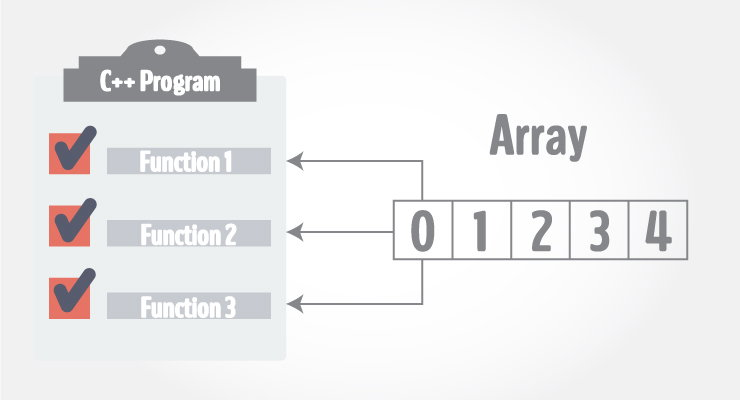# Passing Array to a Function in C++ Programming

In this article, you'll learn to pass an array to a function in C++. You'll learn to pass both one-dimensional and multi-dimensional arrays.Arrays can be passed to a function as an argument. Consider this example to pass one-dimensional array to a function:

## Example 1: Passing One-dimensional Array to a Function

C++ Program to display marks of 5 students by passing one-dimensional array to a function.

``````#include <iostream>
using namespace std;

void display(int marks);

int main()
{
int marks = {88, 76, 90, 61, 69};
display(marks);
return 0;
}

void display(int m)
{
cout << "Displaying marks: "<< endl;

for (int i = 0; i < 5; ++i)
{
cout << "Student "<< i + 1 <<": "<< m[i] << endl;
}
}``````

Output

```Displaying marks:
Student 1: 88
Student 2: 76
Student 3: 90
Student 4: 61
Student 5: 69
```

When an array is passed as an argument to a function, only the name of an array is used as argument.

`display(marks);`

Also notice the difference while passing array as an argument rather than a variable.

`void display(int m);`

The argument `marks` in the above code represents the memory address of first element of array `marks`.

And the formal argument `int m` in function declaration converts to `int* m;`. This pointer points to the same address pointed by the array marks.

That's the reason, although the function is manipulated in the user-defined function with different array name `m`, the original array marks is manipulated.

C++ handles passing an array to a function in this way to save memory and time.

## Passing Multidimensional Array to a Function

Multidimensional array can be passed in similar way as one-dimensional array. Consider this example to pass two dimensional array to a function:

### Example 2: Passing Multidimensional Array to a Function

C++ Program to display the elements of two dimensional array by passing it to a function.

``````#include <iostream>
using namespace std;

void display(int n);

int main()
{
int num = {
{3, 4},
{9, 5},
{7, 1}

};
display(num);

return 0;
}

void display(int n)
{

cout << "Displaying Values: " << endl;
for(int i = 0;  i < 3; ++i)
{
for(int j = 0; j < 2; ++j)
{
cout << n[i][j] << " ";
}
}
}
``````

Output

```Displaying Values:
3 4 9 5 7 1 ```

In the above program, the multi-dimensional array num is passed to the function `display()`.

Inside, `display()` function, the array n (num) is traversed using a nested for loop.

The program uses 2 for loops to iterate over the elements inside a 2-dimensional array. If it were a 3-dimensional array, you should use 3 for loops.

Finally, all elements are printed onto the screen.

Note: Multidimensional array with dimension more than 2 can be passed in similar way as two dimensional array.